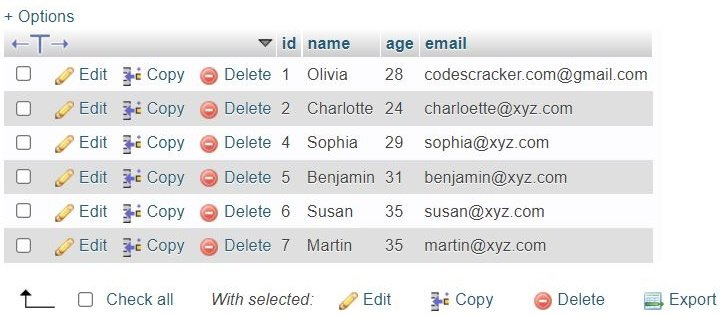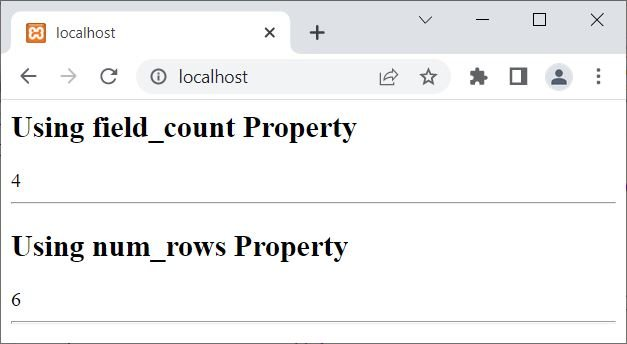# PHP mysqli_result class

The "mysqli_result" class is essential when working with PHP and MySQL databases because it allows developers to interact with the result sets returned from database queries. This class extends the "mysqli" class and provides a set of properties and functions for manipulating and retrieving data from the result set.

## PHP mysqli_result class properties and description

The following is a list of "mysqli_result" class properties and their descriptions.

• current_field: Used to get the current field offset of the result pointer.
• field_count: Used to get the number of fields (columns) available in the result set.
• lengths: Used to get the lengths of columns in the current row in the result set.
• num_rows: Used to get the number of rows.

## PHP mysqli_result Class Methods and Description

Following is a list of "mysqli_result" class functions along with their brief descriptions.

• __construct(): Used to construct a mysqli_result object.
• data_seek(): Used to move the result pointer to an arbitrary row in the result set.
• fetch_all(): Used to fetch all result rows as an associative array, a numeric array, or both.
• fetch_array(): Used to fetch the next row as an associative array, a numeric array, or both.
• fetch_assoc(): Used to fetch the next row as an associative array.
• fetch_column(): Used to fetch a single column from the next row.
• fetch_field_direct(): Used to fetch metadata (data about data) for a single field (column).
• fetch_field(): Used to get the next field (column).
• fetch_fields(): Used to get an array of objects representing the fields.
• fetch_object(): Used to fetch the next row as an object.
• fetch_row(): Used to fetch the next row as an enumerated array.
• field_seek(): Used to set the result pointer to a specified field offset.
• free(): Used to free the memory that is associated with the result set.
• getIterator(): Used to retrieve an external iterator.

## PHP mysqli_result class example

Before creating an example of the mysqli_result class, let's see the table that we are going to use in the example:## PHP mysqli_result class properties example

This example uses the two most famous and commonly used properties of the mysqli_result class in PHP:

```<?php
\$conn = new mysqli("localhost", "root", "", "codescracker");

if(!\$conn->connect_errno)
{
\$stmt = \$conn->prepare("SELECT * FROM customer");
\$stmt->execute();
\$result = \$stmt->get_result();

echo "<h2>Using field_count Property</h2>";
echo \$result->field_count;
echo "<HR>";

echo "<h2>Using num_rows Property</h2>";
echo \$result->num_rows;
echo "<HR>";
}
\$conn->close();
?>```

The output is:## PHP mysqli_result class functions example

This example uses some of the properties of the PHP mysqli_result class:

```<?php
\$conn = new mysqli("localhost", "root", "", "codescracker");

if(!\$conn->connect_errno)
{
\$stmt = \$conn->prepare("SELECT * FROM customer");
\$stmt->execute();
\$result = \$stmt->get_result();

echo "<h2>Using fetch_all() Method</h2>";
print_r(\$result->fetch_all());
echo "<HR>";

echo "<h2>Using fetch_field() Method</h2>";
print_r(\$result->fetch_field());
echo "<HR>";

echo "<h2>Using fetch_fields() Method</h2>";
print_r(\$result->fetch_fields());
echo "<HR>";
}
\$conn->close();
?>```

The output of the above example on the mysqli_result class, based on my current customer table, should be:

```Using fetch_all() Method
Array (  => Array (  => 1  => Olivia  => 28  => codescracker.com@gmail.com )  => Array (  => 2  => Charlotte  => 24  => charloette@xyz.com )  => Array (  => 4  => Sophia  => 29  => sophia@xyz.com )  => Array (  => 5  => Benjamin  => 31  => benjamin@xyz.com )  => Array (  => 6  => Susan  => 35  => susan@xyz.com )  => Array (  => 7  => Martin  => 35  => martin@xyz.com ) )

Using fetch_field() Method
stdClass Object ( [name] => id [orgname] => id [table] => customer [orgtable] => customer [def] => [db] => codescracker [catalog] => def [max_length] => 0 [length] => 6 [charsetnr] => 63 [flags] => 49699 [type] => 3 [decimals] => 0 )

Using fetch_fields() Method
Array (  => stdClass Object ( [name] => id [orgname] => id [table] => customer [orgtable] => customer [def] => [db] => codescracker [catalog] => def [max_length] => 0 [length] => 6 [charsetnr] => 63 [flags] => 49699 [type] => 3 [decimals] => 0 )  => stdClass Object ( [name] => name [orgname] => name [table] => customer [orgtable] => customer [def] => [db] => codescracker [catalog] => def [max_length] => 0 [length] => 120 [charsetnr] => 45 [flags] => 4097 [type] => 253 [decimals] => 0 )  => stdClass Object ( [name] => age [orgname] => age [table] => customer [orgtable] => customer [def] => [db] => codescracker [catalog] => def [max_length] => 0 [length] => 2 [charsetnr] => 63 [flags] => 32768 [type] => 3 [decimals] => 0 )  => stdClass Object ( [name] => email [orgname] => email [table] => customer [orgtable] => customer [def] => [db] => codescracker [catalog] => def [max_length] => 0 [length] => 160 [charsetnr] => 45 [flags] => 4097 [type] => 253 [decimals] => 0 ) )```

PHP Online Test

« Previous Tutorial Next Tutorial »